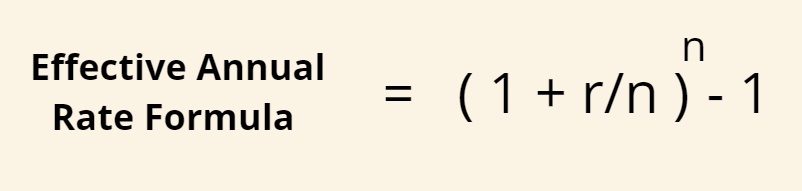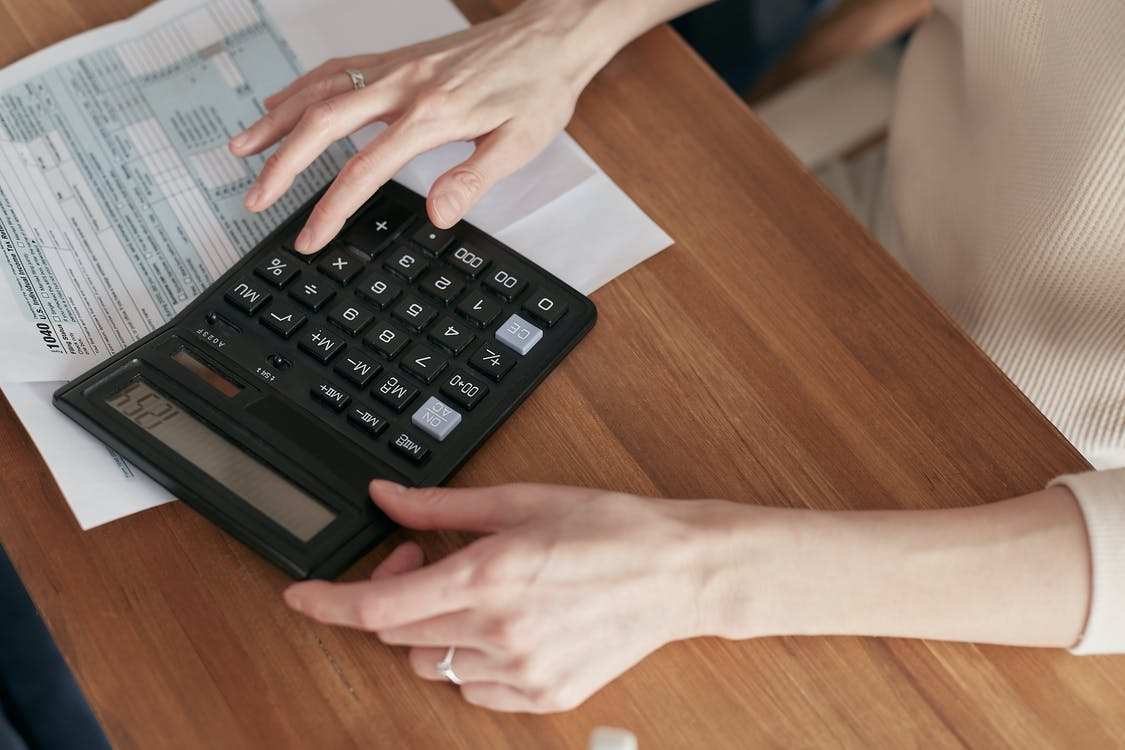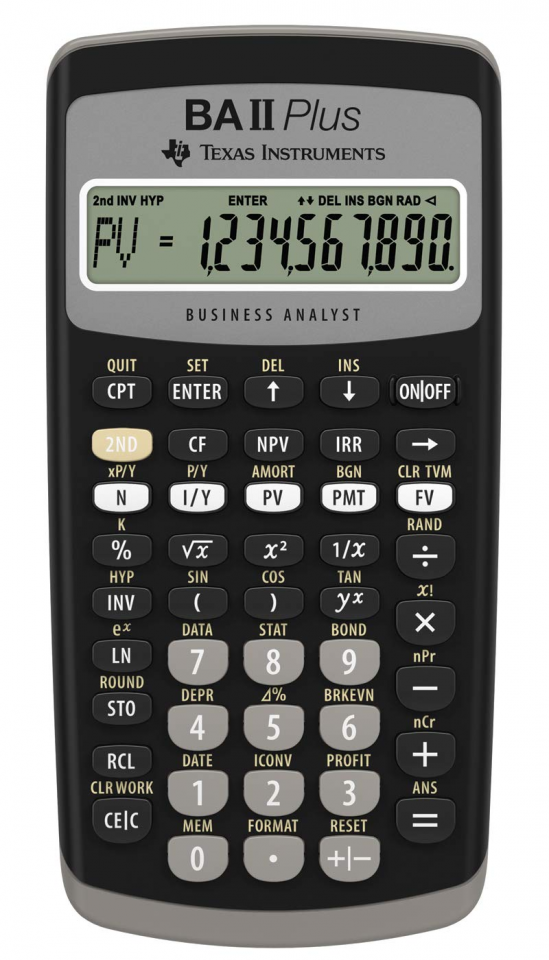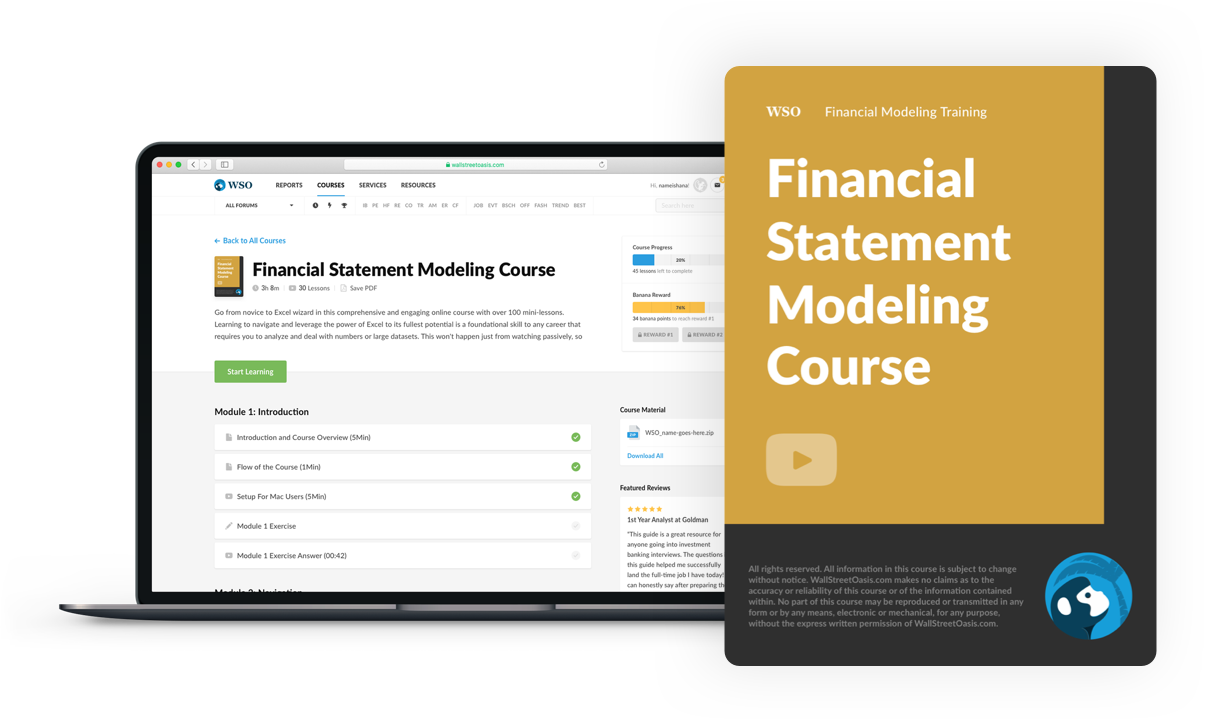# Effective Annual Interest Rate

The actual return on an investment or savings account when the rate is adjusted.

An effective annual interest rate is the actual return on an investment or savings account when the rate is adjusted for compounding over a given period. Simply put, it is the actual percentage rate investor earns or pays in a year, considering compounding.EAR is an effective tool for evaluating interest payable or earnings for a loan/debt or investment. It incorporates the effect of compounding interest over a given time.

Effective Annual Interest Rate (EAR) Formula

The formula contains two major components: the annual interest rate, also called Annual Percentage Return (APR) or Nominal Interest Rate, and the number of compounding periods.

The formula is as follows:

EAR = ((1+i/n)^n)-1

Where,

i = Stated Annual Rate, Nominal Interest Rate, Annual Percentage Return

n = Number of compounding periodsEffective annual rates at different frequencies of compounding nominal
Annual RateFrequency of Compounding
Semi-annualQuarterlyMonthlyDailyContinuous
1%1.003%1.004%1.005%1.005%1.005%
5%5.063%5.095%5.116%5.127%5.127%
10%10.250%10.381%10.471%10.516%10.517%
15%15.563%15.865%16.075%16.180%16.183%
20%21.000%21.511%21.939%22.134%22.140%
30%32.250%33.547%34.489%34.969%34.986%
40%44.000%46.410%48.213%49.150%49.182%
50%56.250%60.181%63.209%64.816%64.872%

The EAR quotes the real interest rate associated with an investment or loan. Again, it has two major components: the nominal interest rate and the number of compounding periods.

The most astonishing feature is that it considers that as the number of compounding periods increases, the effective interest rate gets higher.

## Understanding Effective Annual Interest Rate

Assume that you have two loans, each with a 10% nominal interest rate, one compound annually and the other compound quarterly (four times a year).

Even though both the loans have a stated annual interest rate of 10%, the effective annual interest rate of the loan that compounds four times a year will be higher.

It is also known as the effective interest rate (EIR), annual equivalent rate (AER), or effective rate.

The effective annual rate formula is used to differentiate the actual Internal Rate of Return for an interest rate that may or may not compound multiple times over a given period.

As mentioned before, it can be very well used in comparing different kinds of investment opportunities or loan facilities taken under different capital structures.

But, the concept of compounding has its limits. There is a ceiling to the phenomenon. The limit of compounding is reached when it occurs an infinite number of times. The concept of such recurring compounding is called continuous compounding.

An investment with a 10% interest rate, compounded continuously, will have an effective rate of 10.52%. The formula for continuous compounding is as follows:

e^(i) - 1

Where e is approximately equal to 2.71828. The continuous rate is calculated by raising the e to the power of the interest rate and subtracting one. For this example, the equation will be: e^(10.52%) - 1

## What influences EAR?

Let's demonstrate the concept of compounding with a simple example. Credit card users tend to shop for groceries with a short-term loan from the bank. The bank charges a fee reflected as an interest rate (APR).If the bill is not paid in full every month, one can end up paying interest not only on the principal amount but also on the interest that accrued in the previous month. This concept is called compounding interest. It can be fruitful if one is earning interest, not the other way round.

Since credit card nominal rates do not reflect compounding interest, one needs to calculate the effective annual interest rate to discover the real interest rate on both the interest payment and compounding period.

Similarly, it is true for investments and interest-bearing accounts to evaluate compounding interest earnings or gains.

Lastly, the frequency of compounding determines EAR. The higher the frequency of compounding, the greater the annual equivalent rate (AER). Hence, an account that compounds monthly interest will have greater annual interest than an account that is compounded semi-annually.

## Why is EAR important, and what does it tell?

The effective annual interest rate is an important tool in evaluating the real return on an investment or effective interest rate for a loan. Getting a better understanding of it is crucial for such evaluations.

Say, for example, you are creating a retirement strategy. The aim is to come up with a figure that you need to save monthly to achieve your goal for what you want once you retire or leave the workforce. If the compounding effect of interest is not catered for, you may overestimate or underestimate what you need to save.

Hence, calculating the EAR would give you a better estimate of what needs to be saved every month.It is an important tool because, without it, the borrowers would underestimate the cost of debt or the cost of a loan. And investors may tend to overestimate the actual expected earnings on the investment, such as corporate bonds.

Referring to the second question, a bank may choose to advertise a loan with its nominal and effective rates. But the nominal rate does not suggest compounding the interests that are part of the loan. The EAR is the real percentage return.

This is why it is important to understand the concept of this financial tool. It will assist you in comparing multiple offers.

## Example of EAR or EIR

Consider a bank that offers you two investment opportunities of equal deposits of \$10,000 at 12% and 12.2% stated interest rates.

Investment A compounds the 12% interest daily, and investment B compounds the 12.2% interest on a semiannual basis. The question is, which investment is better?For both investment opportunities, the bank advertised the nominal interest rate. You now have to calculate the effective annual interest rate by adjusting the nominal rate for the number of compounding periods. For both investments, the period is one year.

The formula is

• Effective Annual Interest Rate: (1+(Nominal Rate/No. of compounding periods))^(number of compounding periods) - 1

• For investment A: (1+(12%/365))^(365) - 1 = 12.74%

• For investment B: (1+(12.2%/2)^(2) - 1 = 12.57%

As evident in the example, investment B has a higher stated nominal rate, but the effective annual interest rate is relatively lower than that of investment A.

Even though investment A has a lowered stated interest rate. This is because investment B has fewer compounding periods and hence a lower real rate.

If an investor had to choose between the two investments, he/she would choose the investment with a higher effective annual interest rate.

1. Debt Example

Assume you have \$5000 of the outstanding balance on a credit card with an APR of 20%. A common mistake would be to think that you would pay \$1000 as interest over one year. But the bad news is that a credit card compounds interest daily, so you will need to account for the compounding concept.

Replacing variables with numbers in the equation:

• (1+(0.20/365)^(365) - 1
• (1.0005)^365 - 1
• 1.22 - 1
• 0.22

Hence, the EAR would be 22%. This means that the total interest that you will need to pay is \$1100, not \$1000.2. Investment Example

Though the concept applies in this manner, the terminologies used may vary. Assume that you now want to invest in a savings account with an annual percentage yield (APY) of 15%. Notice that we changed the terminology from a return to yield representing the interest rate effectively.

So now you have invested in a savings account offering an interest rate of 15% compounded semiannually.

• (1+(0.20/2))^(2) - 1
• (1.10)^(2) - 1
• 1.21 - 1
• 0.21

The real percentage rate on the deposit of \$5000 is 21%. You invested \$5000, and you would earn \$1050 in gains instead of \$1000. It helps in calculating the optimal ROI for an investment.

## Steps for calculating Effective Annual Interest Rate (EAR)

A detailed process for calculating the annual equivalent rate using the formula is given below. If you do not have the two components, the annual percentage rate and the number of periods, you can use the APR calculator to find the rate.1. Determine the stated interest rate

The stated return, also called the nominal rate, is stated with the loan or investment proposal. A common example would be: 10% interest charged quarterly.

2. Calculate the number of the compounding period

The compounding period is defined as the number of periods the interest rate compounds over time. Normally, it is monthly, quarterly, or semi-annually. To give you a slightly better idea:

• Daily compounding = 365 compounding periods

• Weekly Compounding = 52 compounding periods

• Bi-weekly compounding = 26 compounding periods

• Monthly compounding = 12 compounding periods

• Quarterly compounding = 4 compounding periods

• Semi-annually = 2 compounding periods

3. Apply the EAR formula

EAR = (1+(i/n))^n - 1

Example: To calculate the effective annual rate of a loan with an annual interest rate of 24%, charged quarterly:

• i = 24%

• n = 4

• (1+(0.24/4))^4 - 1

The EAR is: 26.2%

### Steps for Calculating EAR by Texas BA II Plus Financial CalculatorThis section explains using a financial calculator to calculate the effective rate. This section is of great importance for college students.

1. The first step is to press the 2nd, the first button in the second row, and 2. This would show NOM, also called nominal rate. The nominal rate is the interest rate stated by the bank.

2. Enter the stated nominal rate.

3. Press the down arrow, and you will see EFF and effective rate. It will be zero. The reason why it is zero is that we don't know it yet.

4. Press the down arrow again, and you will see C/Y, representing the number of compounding periods. For example, if the question asks you to compound quarterly, then the compounding per year would be 4.

5. Enter the compounding period.

6. Press the up arrow. The screen will display EFF, and press CPT. This would display the effective annual rate. This is the interest rate after accounting for the compounding effect.

## What interest rate is charged by banks?

Banks tend to advertise nominal interest rates, which are the stated interest rate instead of the effective annual interest rate. This tactic is applied to make consumers believe that they will have to pay lower interest.

For example, for a loan with a stated interest rate of 25% compounded quarterly, the banks would advertise 25% instead of 27.4%.Though this might not be the case when banks are paying interest on the consumer's savings account or deposit, in this case, the effective rate is advertised to attract more customers.

For example, if a deposit with the stated interest rate is 15% compounded monthly, the banks will advertise 16.1% instead of 15%.

Can an effective annual interest rate be lowered by improving credit?

Lenders, majorly banks, determine interest rates based on one's creditworthiness, and the lower the credit score, the higher the real rate can be. One must review his/her credit history to work on credit. This will improve the chances of scoring a lower rate when applying for a loan.